# PREDICTING CHEMICAL REACTIONS Single displacement Reactions Mgs Zn

• Slides: 27PREDICTING CHEMICAL REACTIONS: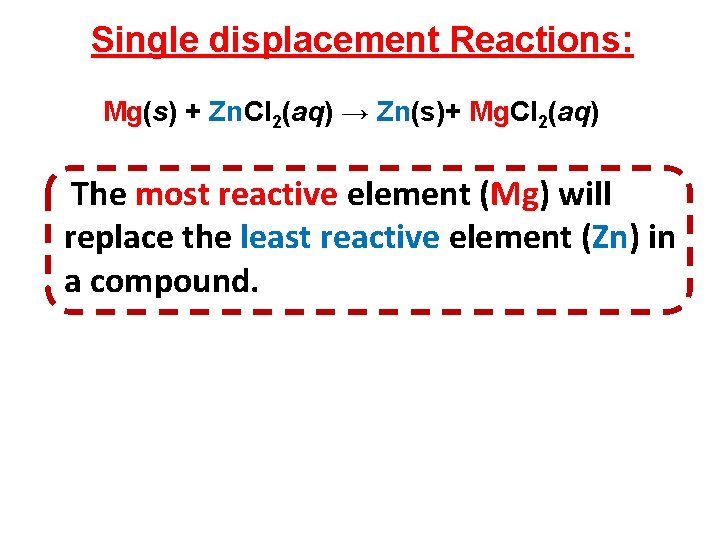Single displacement Reactions: Mg(s) + Zn. Cl 2(aq) → Zn(s)+ Mg. Cl 2(aq) The most reactive element (Mg) will replace the least reactive element (Zn) in a compound.Single displacement Reactions: Mg(s) + Zn. Cl 2(aq) → Zn(s)+ Mg. Cl 2(aq) The most reactive element (Mg) will replace the least reactive element (Zn) in a compound. See Activity Series of metals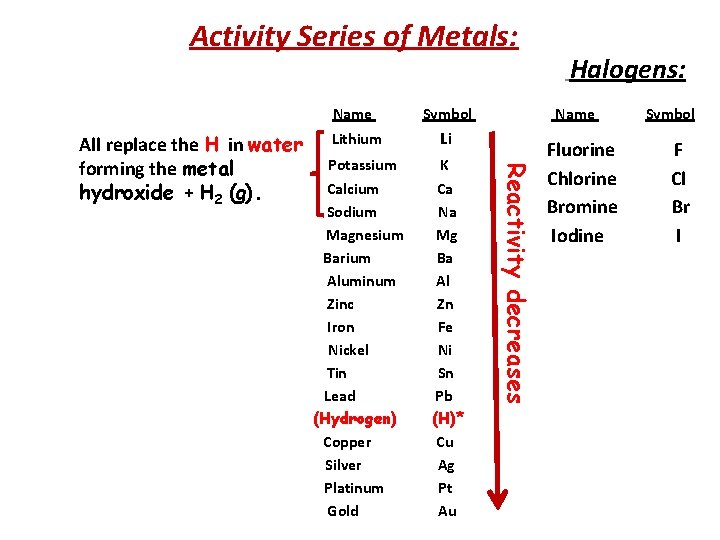Activity Series of Metals: Symbol Lithium Li Potassium Calcium Sodium Magnesium Barium Aluminum Zinc Iron Nickel Tin Lead (Hydrogen) Copper Silver Platinum Gold K Ca Na Mg Ba Al Zn Fe Ni Sn Pb (H)* Cu Ag Pt Au Name Reactivity decreases All replace the H in water forming the metal hydroxide + H 2 (g). Name Halogens: Fluorine Chlorine Bromine Iodine Symbol F Cl Br IEXERCISE: Predict if the following reactions will occur. If yes, complete the chemical balanced equation : 1) Ca(s) + Zn. Cl 2(aq) 2) Fe(s) + KOH(aq) 3) HCl(aq) + Zn(s) 4) HCl(aq) + Cu(s) → →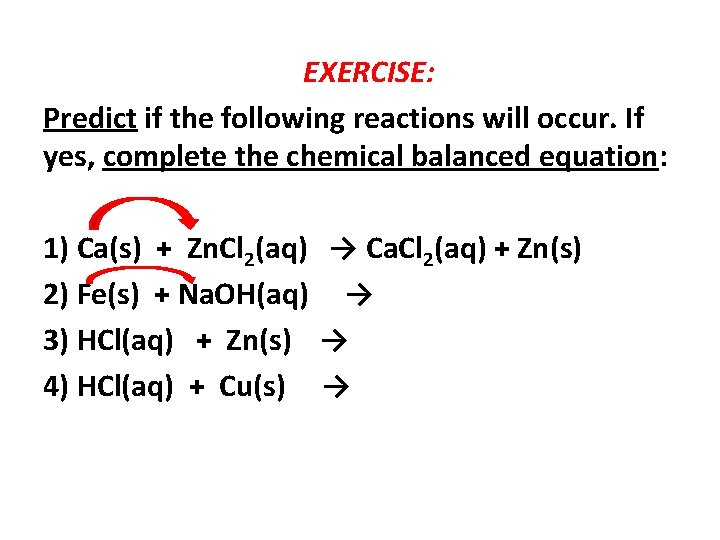EXERCISE: Predict if the following reactions will occur. If yes, complete the chemical balanced equation: 1) Ca(s) + Zn. Cl 2(aq) → Ca. Cl 2(aq) + Zn(s) 2) Fe(s) + Na. OH(aq) → 3) HCl(aq) + Zn(s) → 4) HCl(aq) + Cu(s) →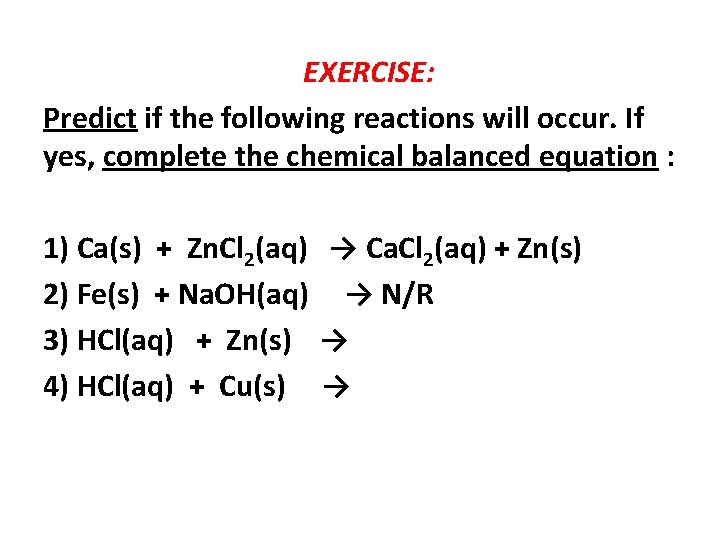EXERCISE: Predict if the following reactions will occur. If yes, complete the chemical balanced equation : 1) Ca(s) + Zn. Cl 2(aq) → Ca. Cl 2(aq) + Zn(s) 2) Fe(s) + Na. OH(aq) → N/R 3) HCl(aq) + Zn(s) → 4) HCl(aq) + Cu(s) →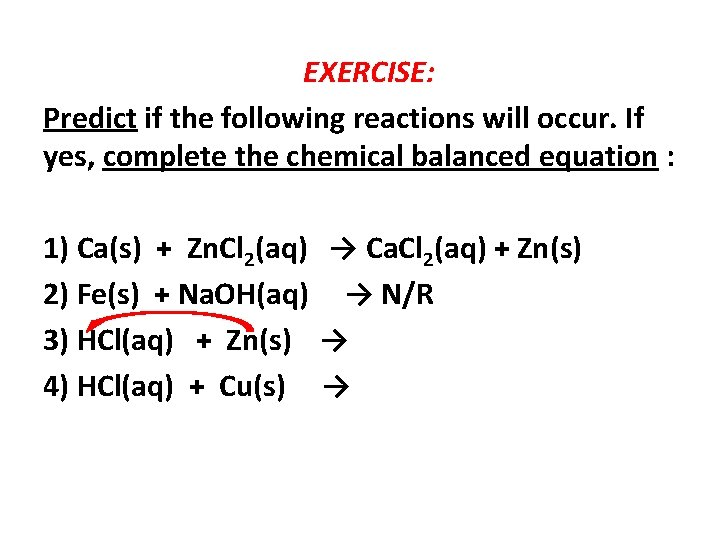EXERCISE: Predict if the following reactions will occur. If yes, complete the chemical balanced equation : 1) Ca(s) + Zn. Cl 2(aq) → Ca. Cl 2(aq) + Zn(s) 2) Fe(s) + Na. OH(aq) → N/R 3) HCl(aq) + Zn(s) → 4) HCl(aq) + Cu(s) →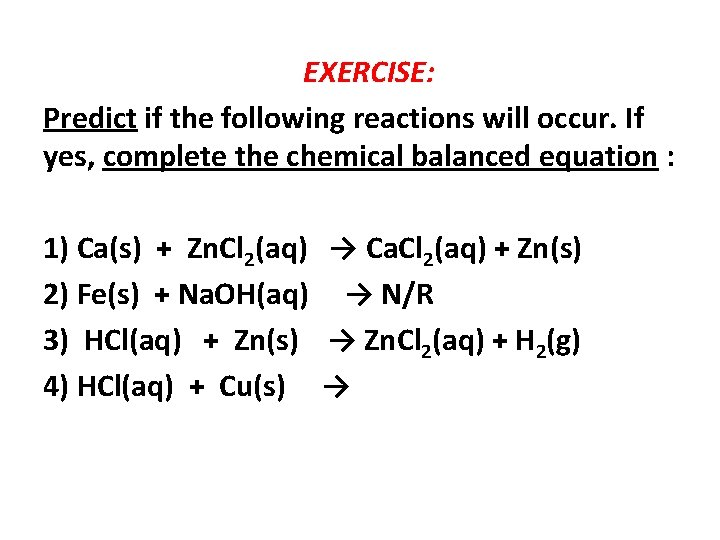EXERCISE: Predict if the following reactions will occur. If yes, complete the chemical balanced equation : 1) Ca(s) + Zn. Cl 2(aq) → Ca. Cl 2(aq) + Zn(s) 2) Fe(s) + Na. OH(aq) → N/R 3) HCl(aq) + Zn(s) → Zn. Cl 2(aq) + H 2(g) 4) HCl(aq) + Cu(s) →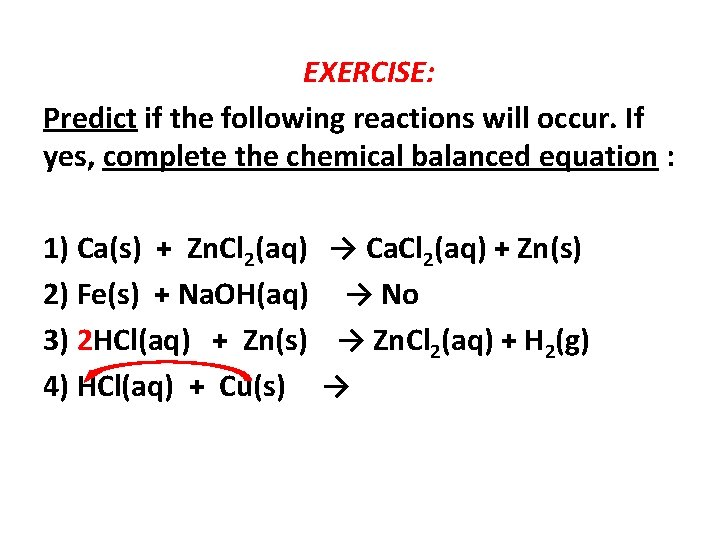EXERCISE: Predict if the following reactions will occur. If yes, complete the chemical balanced equation : 1) Ca(s) + Zn. Cl 2(aq) → Ca. Cl 2(aq) + Zn(s) 2) Fe(s) + Na. OH(aq) → No 3) 2 HCl(aq) + Zn(s) → Zn. Cl 2(aq) + H 2(g) 4) HCl(aq) + Cu(s) →EXERCISE: Predict if the following reactions will occur. If yes, complete the chemical balanced equation : 1) Ca(s) + Zn. Cl 2(aq) → Ca. Cl 2(aq) + Zn(s) 2) Fe(s) + Na. OH(aq) → No 3) 2 HCl(aq) + Zn(s) → Zn. Cl 2(aq) + H 2(g) 4) HCl(aq) + Cu(s) → N/R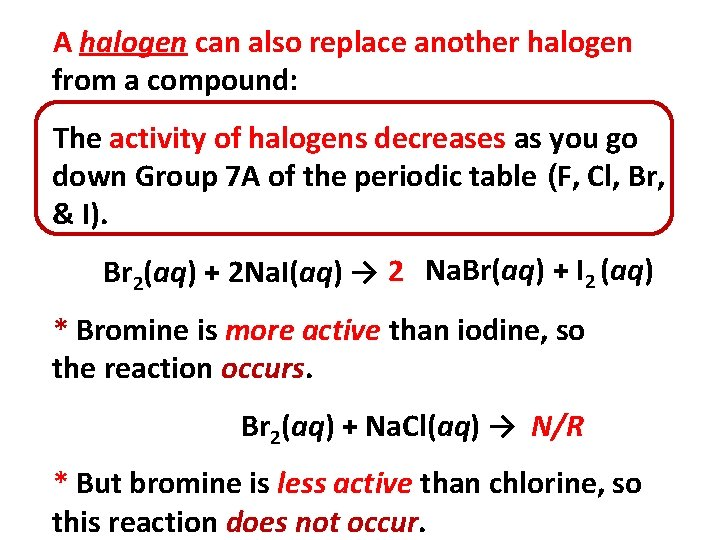A halogen can also replace another halogen from a compound: The activity of halogens decreases as you go down Group 7 A of the periodic table (F, Cl, Br, & I). Br 2(aq) + 2 Na. I(aq) → 2 Na. Br(aq) + I 2 (aq) * Bromine is more active than iodine, so the reaction occurs. Br 2(aq) + Na. Cl(aq) → N/R * But bromine is less active than chlorine, so this reaction does not occur.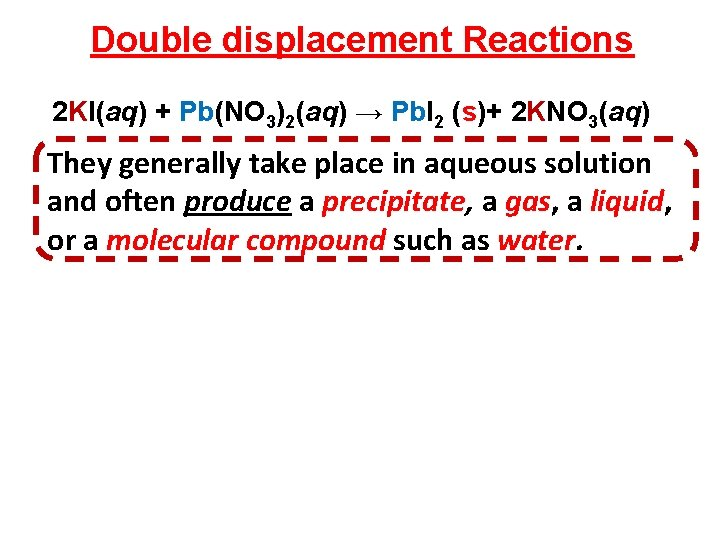Double displacement Reactions 2 KI(aq) + Pb(NO 3)2(aq) → Pb. I 2 (s)+ 2 KNO 3(aq) They generally take place in aqueous solution and often produce a precipitate, a gas, a liquid, or a molecular compound such as water.Double displacement Reactions 2 KI(aq) + Pb(NO 3)2(aq) → Pb. I 2 (s)+ 2 KNO 3(aq) They generally take place in aqueous solution and often produce a precipitate, a gas, a liquid, or a molecular compound such as water. Examples: KCl(aq) + Ag. NO 3(aq) → Na. OH(aq) + HCl(aq) → HCI(aq) + Ca. CO 3(s) →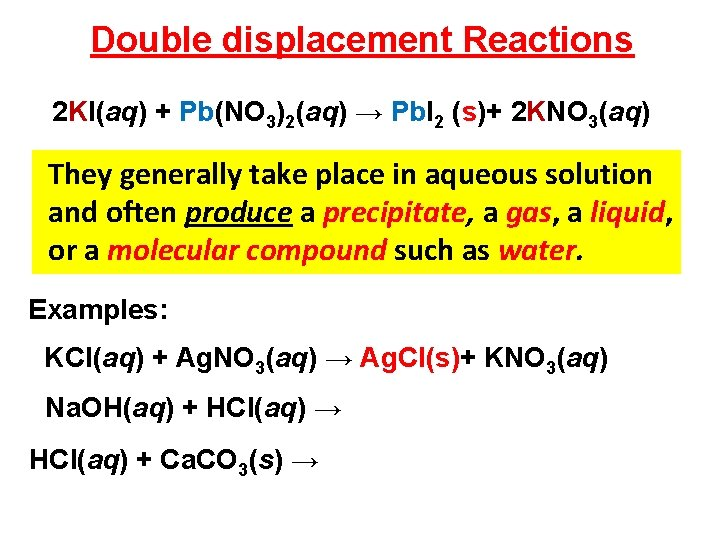Double displacement Reactions 2 KI(aq) + Pb(NO 3)2(aq) → Pb. I 2 (s)+ 2 KNO 3(aq) They generally take place in aqueous solution and often produce a precipitate, a gas, a liquid, or a molecular compound such as water. Examples: KCl(aq) + Ag. NO 3(aq) → Ag. Cl(s)+ KNO 3(aq) Na. OH(aq) + HCl(aq) → HCI(aq) + Ca. CO 3(s) →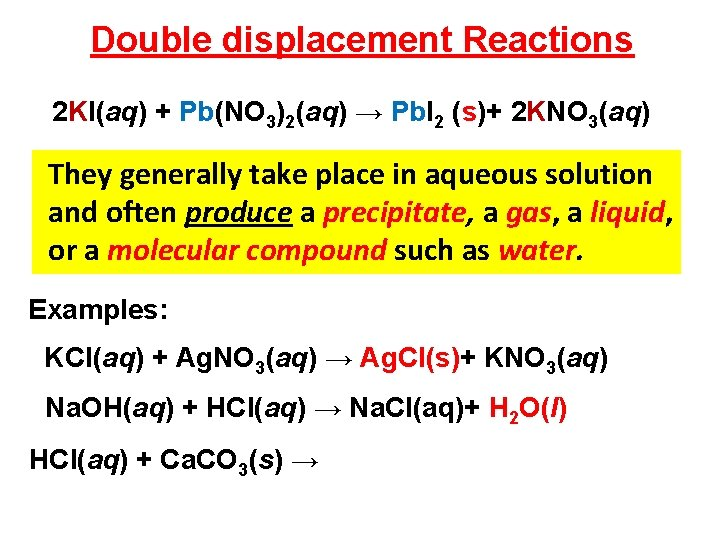Double displacement Reactions 2 KI(aq) + Pb(NO 3)2(aq) → Pb. I 2 (s)+ 2 KNO 3(aq) They generally take place in aqueous solution and often produce a precipitate, a gas, a liquid, or a molecular compound such as water. Examples: KCl(aq) + Ag. NO 3(aq) → Ag. Cl(s)+ KNO 3(aq) Na. OH(aq) + HCl(aq) → Na. Cl(aq)+ H 2 O(l) HCI(aq) + Ca. CO 3(s) →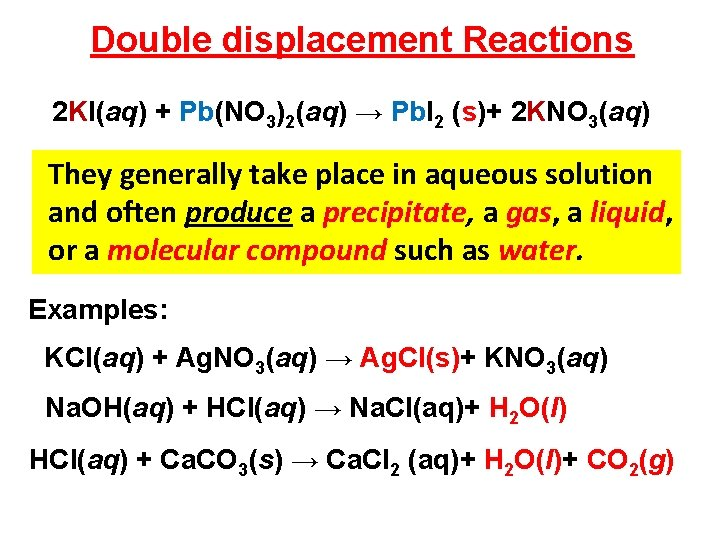Double displacement Reactions 2 KI(aq) + Pb(NO 3)2(aq) → Pb. I 2 (s)+ 2 KNO 3(aq) They generally take place in aqueous solution and often produce a precipitate, a gas, a liquid, or a molecular compound such as water. Examples: KCl(aq) + Ag. NO 3(aq) → Ag. Cl(s)+ KNO 3(aq) Na. OH(aq) + HCl(aq) → Na. Cl(aq)+ H 2 O(l) HCI(aq) + Ca. CO 3(s) → Ca. CI 2 (aq)+ H 2 O(l)+ CO 2(g)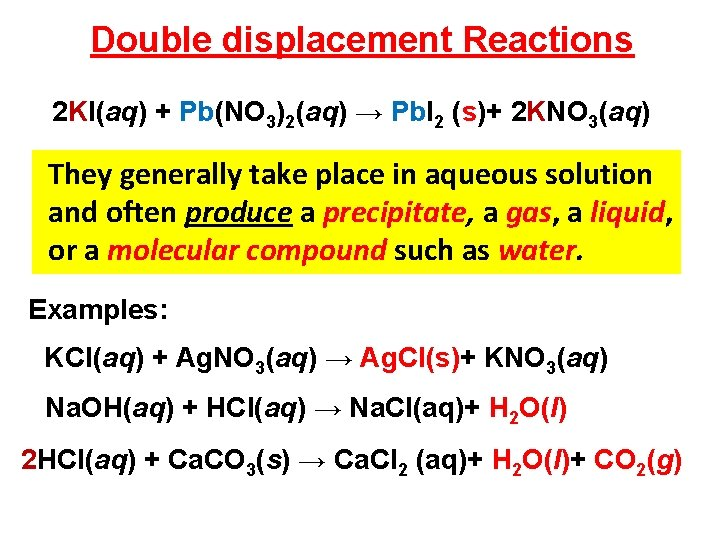Double displacement Reactions 2 KI(aq) + Pb(NO 3)2(aq) → Pb. I 2 (s)+ 2 KNO 3(aq) They generally take place in aqueous solution and often produce a precipitate, a gas, a liquid, or a molecular compound such as water. Examples: KCl(aq) + Ag. NO 3(aq) → Ag. Cl(s)+ KNO 3(aq) Na. OH(aq) + HCl(aq) → Na. Cl(aq)+ H 2 O(l) 2 HCI(aq) + Ca. CO 3(s) → Ca. CI 2 (aq)+ H 2 O(l)+ CO 2(g)Exercise: Predict and complete: 1) Zn(s) + Cu(NO 3)2(aq) → 2) Cu(s) + Zn(NO 3)2(aq) → 3) Na. OH(aq) + Zn(NO 3)2(aq) → 4) KCl(aq) + Mg(NO 3)2(aq) →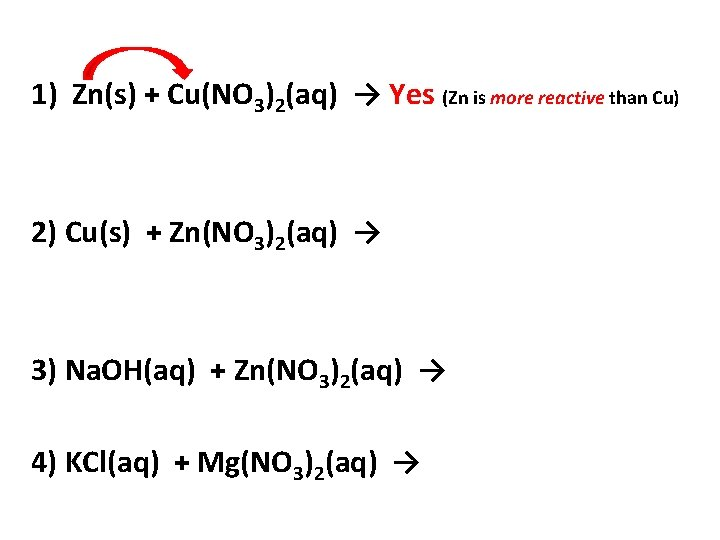1) Zn(s) + Cu(NO 3)2(aq) → Yes (Zn is more reactive than Cu) 2) Cu(s) + Zn(NO 3)2(aq) → 3) Na. OH(aq) + Zn(NO 3)2(aq) → 4) KCl(aq) + Mg(NO 3)2(aq) →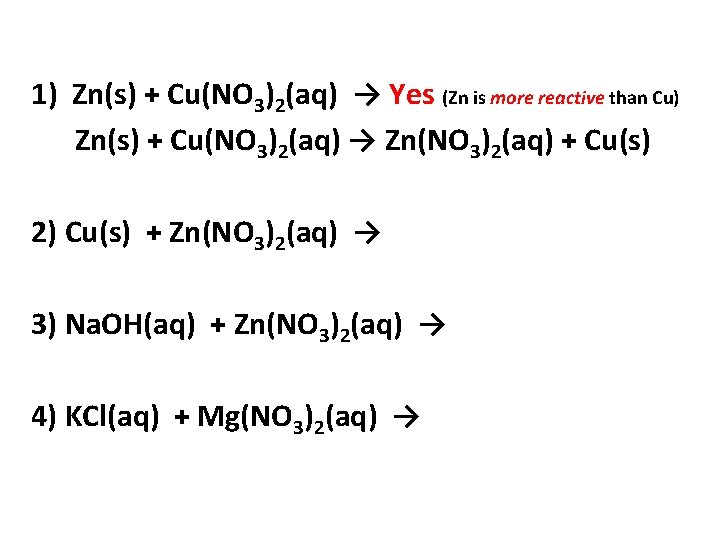1) Zn(s) + Cu(NO 3)2(aq) → Yes (Zn is more reactive than Cu) Zn(s) + Cu(NO 3)2(aq) → Zn(NO 3)2(aq) + Cu(s) 2) Cu(s) + Zn(NO 3)2(aq) → 3) Na. OH(aq) + Zn(NO 3)2(aq) → 4) KCl(aq) + Mg(NO 3)2(aq) →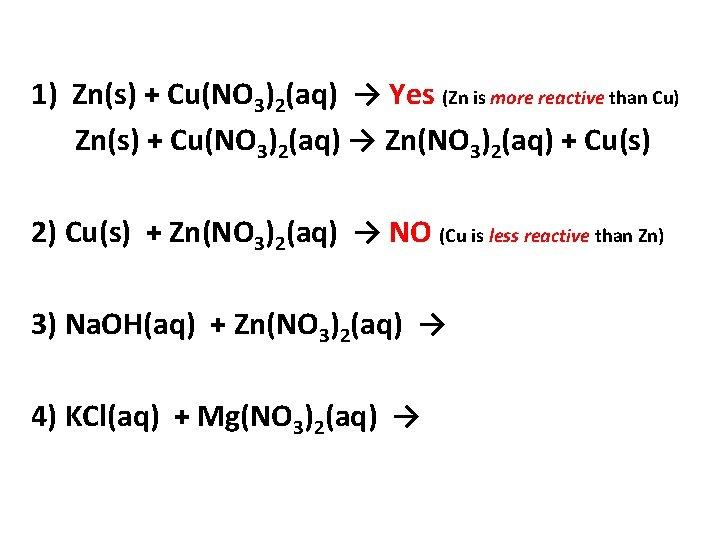1) Zn(s) + Cu(NO 3)2(aq) → Yes (Zn is more reactive than Cu) Zn(s) + Cu(NO 3)2(aq) → Zn(NO 3)2(aq) + Cu(s) 2) Cu(s) + Zn(NO 3)2(aq) → NO (Cu is less reactive than Zn) 3) Na. OH(aq) + Zn(NO 3)2(aq) → 4) KCl(aq) + Mg(NO 3)2(aq) →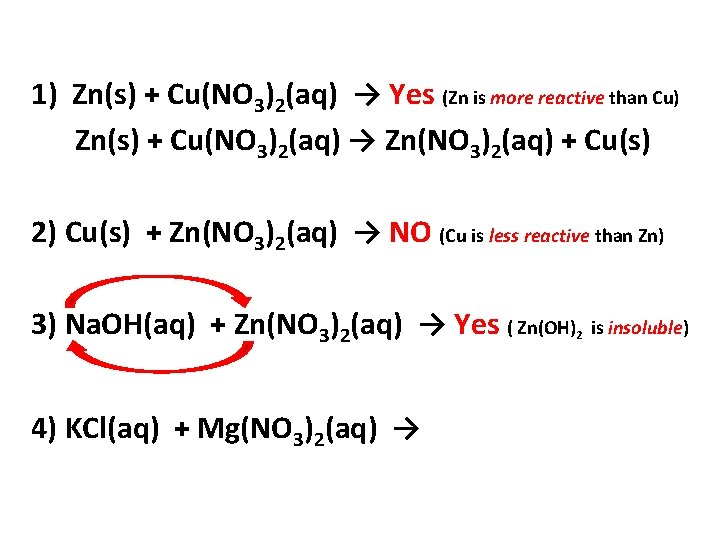1) Zn(s) + Cu(NO 3)2(aq) → Yes (Zn is more reactive than Cu) Zn(s) + Cu(NO 3)2(aq) → Zn(NO 3)2(aq) + Cu(s) 2) Cu(s) + Zn(NO 3)2(aq) → NO (Cu is less reactive than Zn) 3) Na. OH(aq) + Zn(NO 3)2(aq) → Yes ( Zn(OH)2 is insoluble) 4) KCl(aq) + Mg(NO 3)2(aq) →1) Zn(s) + Cu(NO 3)2(aq) → Yes (Zn is more reactive than Cu) Zn(s) + Cu(NO 3)2(aq) → Zn(NO 3)2(aq) + Cu(s) 2) Cu(s) + Zn(NO 3)2(aq) → NO (Cu is less reactive than Zn) 3) Na. OH(aq) + Zn(NO 3)2(aq) → Yes ( Zn(OH)2 is insoluble) Na. OH(aq) + Zn(NO 3)2(aq) → Na. NO 3(aq) + Zn(OH)2(s) 4) KCl(aq) + Mg(NO 3)2(aq) →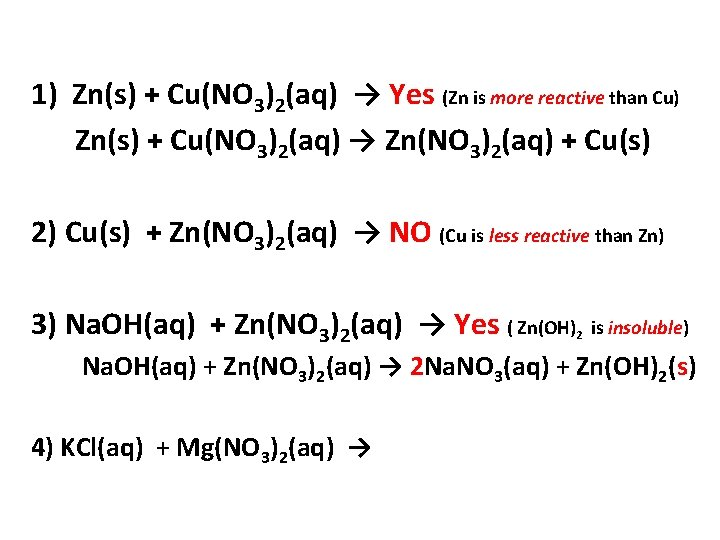1) Zn(s) + Cu(NO 3)2(aq) → Yes (Zn is more reactive than Cu) Zn(s) + Cu(NO 3)2(aq) → Zn(NO 3)2(aq) + Cu(s) 2) Cu(s) + Zn(NO 3)2(aq) → NO (Cu is less reactive than Zn) 3) Na. OH(aq) + Zn(NO 3)2(aq) → Yes ( Zn(OH)2 is insoluble) Na. OH(aq) + Zn(NO 3)2(aq) → 2 Na. NO 3(aq) + Zn(OH)2(s) 4) KCl(aq) + Mg(NO 3)2(aq) →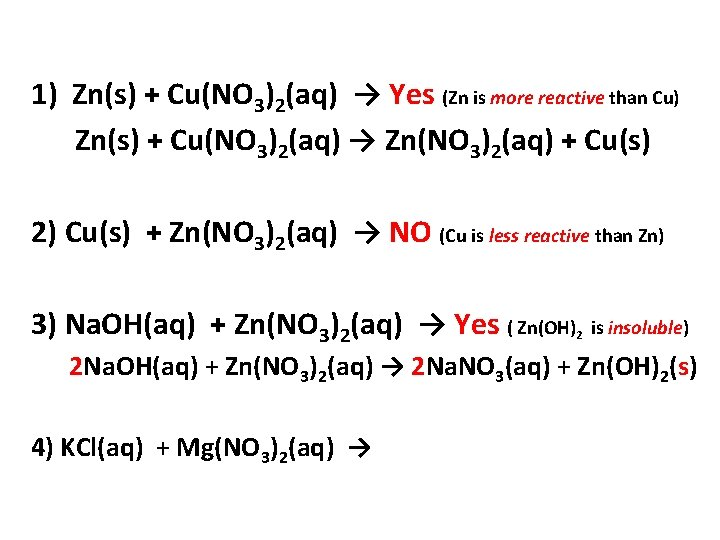1) Zn(s) + Cu(NO 3)2(aq) → Yes (Zn is more reactive than Cu) Zn(s) + Cu(NO 3)2(aq) → Zn(NO 3)2(aq) + Cu(s) 2) Cu(s) + Zn(NO 3)2(aq) → NO (Cu is less reactive than Zn) 3) Na. OH(aq) + Zn(NO 3)2(aq) → Yes ( Zn(OH)2 is insoluble) 2 Na. OH(aq) + Zn(NO 3)2(aq) → 2 Na. NO 3(aq) + Zn(OH)2(s) 4) KCl(aq) + Mg(NO 3)2(aq) →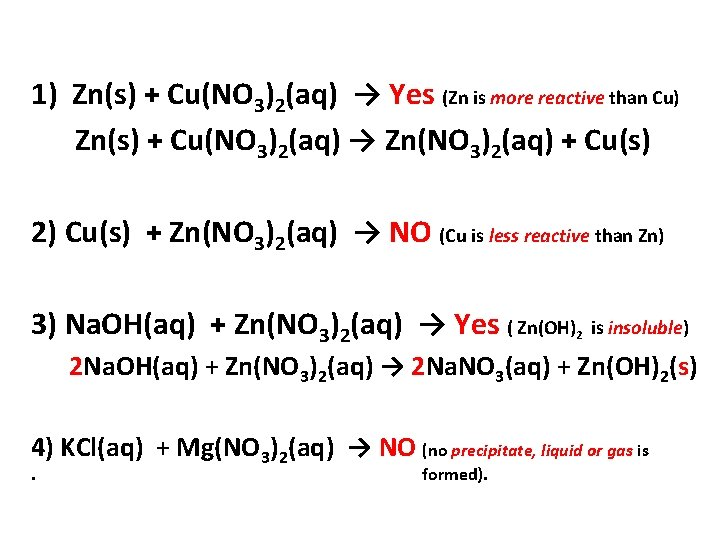1) Zn(s) + Cu(NO 3)2(aq) → Yes (Zn is more reactive than Cu) Zn(s) + Cu(NO 3)2(aq) → Zn(NO 3)2(aq) + Cu(s) 2) Cu(s) + Zn(NO 3)2(aq) → NO (Cu is less reactive than Zn) 3) Na. OH(aq) + Zn(NO 3)2(aq) → Yes ( Zn(OH)2 is insoluble) 2 Na. OH(aq) + Zn(NO 3)2(aq) → 2 Na. NO 3(aq) + Zn(OH)2(s) 4) KCl(aq) + Mg(NO 3)2(aq) → NO (no precipitate, liquid or gas is. formed).